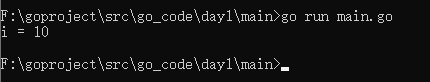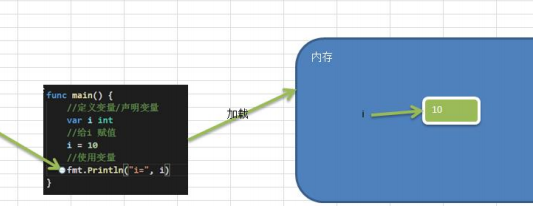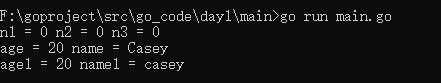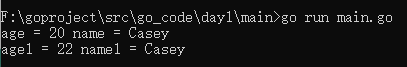# Why do you need variables

A program is a world, and variables are the basic unit of the program.
Regardless of which high-level programming language is used to write a program, variables are the basic components of the program. For example, a schematic diagram:sum and sub in the above figure are variables.

# Introduction to variables

## The concept of variables

Variables are equivalent to the representation of a data storage space in memory. Variables can be regarded as the house number of a room. The room can be found by the house number. In the same way, variables (values) can be accessed through variables.

## Steps to use variables

1. Declare variables (also called: define variables)
2. Non-variable assignment
3. Use variables

# Variable quick start case

Look at the following program:

``````package main
import "fmt"

func main() {

//定义变量
var i int
//给变量i赋值
i = 10
//使用变量
fmt.Println("i =",i)
}
``````

Output result:# Precautions for using variables

• Variable represents a storage area in memory
• This area has its own name (variable name) and type (data type)
diagram:## Three ways to use Golang variables

(1) Specify the variable type, if no value is assigned after declaration, use the default value

``````func main() {

//int 的默认值是0
var i int
fmt.Println("i =",i)
}
``````

(2) Judge the variable type based on the value

``````
func main() {

var i = 1.11
fmt.Println("i =",i)
}
``````

(3) Omit var, doctrine: The variable on the left side should not have been declared, otherwise it will cause a compilation error.

``````func main() {

//等价于var i int i = 10
i := 10
fmt.Println("i =",i)
}
``````

## Multivariate declaration

In programming, sometimes we need to declare multiple variables at once, and Golang also provides such a syntax.

1. Declare multiple variables at once

``````package main
import "fmt"

func main() {

//一次性声明多个变量方式1
var n1, n2, n3 int
fmt.Println("n1 =",n1,"n2 =",n2,"n3 =",n3)

//一次性声明多个变量方式2
var age, name = 20, "Casey"
fmt.Println("age =",age,"name =",name)

//一次性声明多个变量方式3，可以使用各类型推导
age1, name1 := 20,"casey"
fmt.Println("age1 =",age1,"name1 =",name1)
}
``````

Running results:2. Declare multiple global variables
at once. In go, the variables defined outside the function are global variables.
for example

``````package main
import "fmt"

//定义全局变量
var age = 20
var name = "Casey"
//上面的声明方式，也可以改成一次性声明
var(
age1 = 22
name1 = "Casey"
)
func main() {

fmt.Println("age =",age,"name =",name)
fmt.Println("age1 =",age1,"name1 =",name1)
}
``````

Note on running results:

• Variables cannot have the same name in the same scope (in a function or in a code block).
• Variable = variable name + value + data type, the three elements of the variable.
• If the Golang variable is not assigned an initial value, the compiler will use the default value. The default value of int is 0, the default value of string is an empty string, the decimal default is 0, and the Boolean value defaults to false.

# Variable declaration, initialization, assignment

## Declare variable

``````基本语法：var 变量名 数据类型
var i int这就声明了一个变量，变量名是a，数据类型为int型
var num float32  声明了一个单精度类型的小数，变量名是num
``````

## Initialize variables

``````在声明变量的时候，就给值
var a int = 45 这就是初始化变量a

var b = 45
``````

## Assign a value to a variable

``````若先声明了变量：var num int //默认值为0

``````

### Guess you like

Origin blog.csdn.net/weixin_44736475/article/details/113858029
Recommended
Ranking
Daily